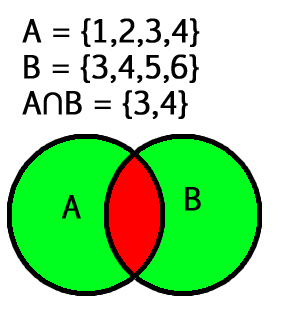# Intersection() function Python

Intersection of two given sets is the largest set which contains all the elements that are common to both the sets. Intersection of two given sets A and B is a set which consists of all the elements which are common to both A and B.Examples:

```Input: Let set A = {2, 4, 5, 6}
and set B = {4, 6, 7, 8}
Output: {4,6}
Explanation: Taking the common elements in both the sets,
we get {4,6} as the intersection of both the sets.
```

Syntax:

set1.intersection(set2, set3, set4….)
In parameters, any number of sets can be given

Return value:

The intersection() function returns a set, which has the intersection of all sets(set1, set2, set3…) with set1.
It returns a copy of set1 only if no parameter is passed.

Below is the Python3 implementation of the above approach:

 `# Python3 program for intersection() function ` ` `  `set1 ``=` `{``2``, ``4``, ``5``, ``6``}  ` `set2 ``=` `{``4``, ``6``, ``7``, ``8``}  ` `set3 ``=` `{``4``,``6``,``8``} ` ` `  `# union of two sets ` `print``(``"set1 intersection set2 : "``, set1.intersection(set2)) ` ` `  `# union of three sets ` `print``(``"set1 intersection set2 intersection set3 :"``, set1.intersection(set2,set3)) `

Output:

```set1 intersection set2 :  {4, 6}
set1 intersection set2 intersection set3 : {4, 6}
```

Practical applications:
In most of the probability problems, the concept of intersection of sets is needed.

My Personal Notes arrow_drop_upCheck out this Author's contributed articles.

If you like GeeksforGeeks and would like to contribute, you can also write an article using contribute.geeksforgeeks.org or mail your article to contribute@geeksforgeeks.org. See your article appearing on the GeeksforGeeks main page and help other Geeks.

Please Improve this article if you find anything incorrect by clicking on the "Improve Article" button below.

Article Tags :
Practice Tags :

2

Please write to us at contribute@geeksforgeeks.org to report any issue with the above content.